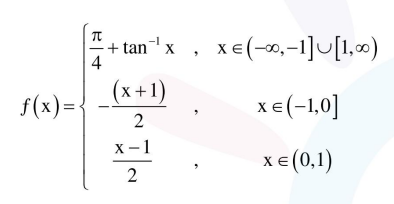# Solve this

Question:

The function $f(x)=\left\{\begin{array}{l}\frac{\pi}{4}+\tan ^{-1} x,|x| \leq 1 \\ \frac{1}{2}(|x|-1),|x|>1\end{array}\right.$ is :

1. continuous on $\mathrm{R}-\{1\}$ and differentiable on

$\mathrm{R}-\{-1,1\}$.

2. both continuous and differentiable on

$R-\{-1\}$.

3. continuous on $R-\{-1\}$ and differentiable on

$\mathrm{R}-\{-1,1\}$.

4. both continuous and differentiable on

$\mathrm{R}-\{1\}$

Correct Option: 1

Solution:for continuity at $\mathrm{x}=-1$

L.H.L. $=\frac{\pi}{4}-\frac{\pi}{4}=0$

R.H.L. $=0$

so, continuous at $x=-1$

for continuity at $x=1$

L.H.L. $=0$

R.H.L. $=\frac{\pi}{4}+\frac{\pi}{4}=\frac{\pi}{2}$

so, not continuous at $x=1$

For differentiability at $x=-1$

L.H.D. $=\frac{1}{1+1}=\frac{1}{2}$

R.H.D. $=-\frac{1}{2}$

so, non differentiable at $x=-1$